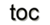Next: 7.4 Performance Analysis Up: 7. A Generic Scientific Previous: 7.2 The Generic Functor

Subsections

As stated, additional generic concepts are available within the GSSE  to further ease application design. They all deal with interfaces to various areas of scientific computing.

## 7.3.1 Generic Solver Interface, GSI

Access to algebraic solver systems requires different mechanisms, if used for a wide variety of discretization schemes. Therefore the GSSE  provides a generic solver interface which is based on the introduced traversal and quantity accessors and linearization mechanisms.

The most important parts of the GSI are given in the following short code snippets. First, the compile or run-time specification is illustrated:The generic matrix wrapper uses a bijective connection of the assembled quantities and the corresponding domain to provide easy access, where msi represents the matrix-solver interface: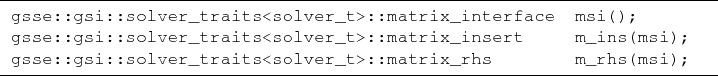The given quantity accessors are then connected to the solver interface, illustrated in the following: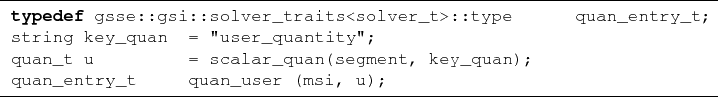thereby creating a quantity u which is directly connected to the matrix-solver interface msi by the object quan_user. The matrix-solver interface also handles the write-back of the solved quantity value. Each quantity is attributed to a segment within a domain.

The use of the final solution procedure with different options is demonstrated in the next code snippet.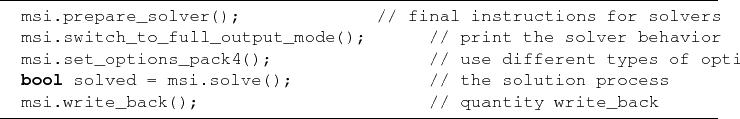Several algebraic solver software packages are accessible, such as the self-developed direct and iterative solvers  and solver packages like Trilinos  and PetSc , thereby providing a multitude of different solving procedures.

## 7.3.2 Generic Orthogonal Range Queries

Point location is a necessary requirement for efficient algorithms, such as interpolation, intersection tests, or boundary operators, e.g., for TCAD . Different types of point location mechanisms can be summarized by the concept of orthogonal range queries. The interface of generic orthogonal range queries presented here can be used completely orthogonally, which means that different range query algorithms and libraries can be used. The example is based on the range query concepts and libraries of Mauch ,

• Kd-trees, quadtrees and octrees: The kd-tree outperforms the octree methods in most cases. In addition to the higher execution times, octree methods also have several times the memory footprint of a kd-tree. This fact is due to the differences in how these methods organize their records . Finally, kd-trees can be used as a generic range query method in a wide range of applications.

• Cell and sparse cell methods: Sparse cell arrays have execution times that are almost as low as dense cell arrays. Therefore, if the dense cell array has many empty cells, the sparse cell method reduces the amount of required memory storage without affecting the execution time.

Due to the performance dependencies of orthogonal range queries, based on many parameters, such as the dimension of the stored record, the number of records, their distribution, and the query range, it is of utmost importance to have a wide variety of range query modules available. Finally, there is no best method  for different application scenarios.

The application by the GSSE for all different types of range query methods is always given by: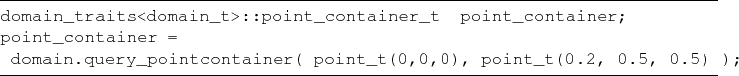## 7.3.3 Generic File Interface, GFI

The file interface is implemented by means of the fiber bundle data model [1,136]. By the implementation of fiber bundle within GSSE, the data structures map directly to the hierarchical file format. An example is given by a file resulting from a numerical simulation which has to encompass the time-dependent output of Maxwell's equations, such as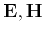. These fields live on different skeletons of the grid:

• Primary mesh edge fields: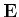• Primary mesh face fields: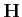Using the F5 layer the following output information is obtained:

/T=1.0/GSSE/Points Group
/T=1.0/GSSE/Points/Cartesian Group
/T=1.0/GSSE/Points/Cartesian/Positions Dataset {40457}
/T=1.0/GSSE/Edges Group
/T=1.0/GSSE/Edges/Points Group
/T=1.0/GSSE/Edges/Points/Positions Dataset {81317}
/T=1.0/GSSE/Edges/Cartesian/E Dataset {81317}
/T=1.0/GSSE/Faces Group
/T=1.0/GSSE/Faces/Points Group
/T=1.0/GSSE/Faces/Points/Positions Dataset {53965}
/T=1.0/GSSE/Faces/Cartesian/H Dataset {53965}

The file structure provides a grouping of the different simulation fields according to the topological relations of their skeletons, given here by the points, edges, and faces for one time step. A Cartesian coordinate representation is used.

## 7.3.4 Finite Element Components

The assembly procedure of finite elements requires additional steps for efficient implementation, as given in Section 2.3. Element-wise assembly is typically used in the finite element method, where all cells are traversed and for each of the cells a local matrix is calculated. This matrix introduces coupling factors between the basis functions. As each basis function is mapped to an element of the underlying cell complex, couplings between functions can be seen as couplings between values on elements. The local matrix entries are written into the global matrix according to a global vertex/cell-numbering scheme. The following source snippet presents the important operations for finite element applications: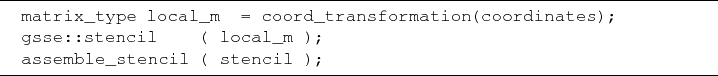As stated in Section 2.3, the key for an efficient realization of the finite element method is the transformation of a reference element in a normalized coordinate system (Equation 2.38 - Equation 2.40). To support this operation, GSSE contains a coordination transformation algorithm. C++'s partial specialization feature is used, as given in the following source snippet for a three-dimensional simplex cell.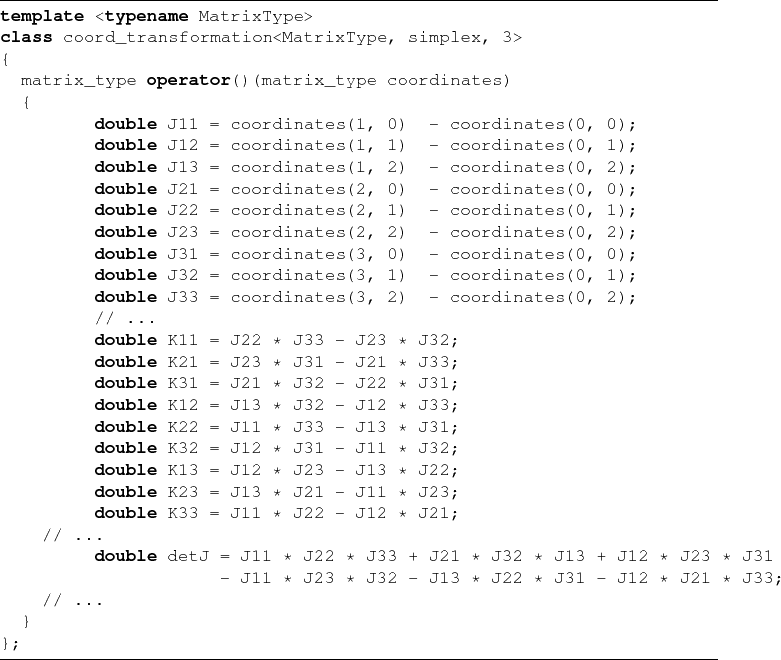The following pre-calculated element matrices for the Laplace operator are used , which are implemented for two- and three-dimensional simplex and cuboid cells and partially given in the following source snippet for a three-dimensional simplex example: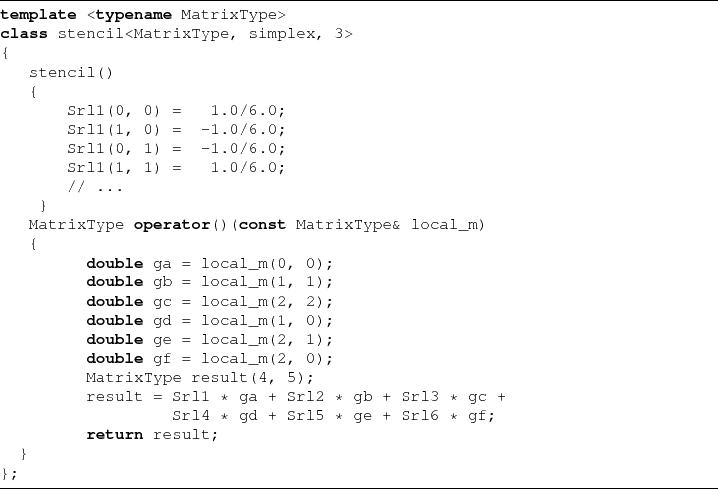By utilizing the GSI, the assembly of the local stencil matrix is then given by the following algorithm, where the index_list stores the transformation of local node indices to global ones: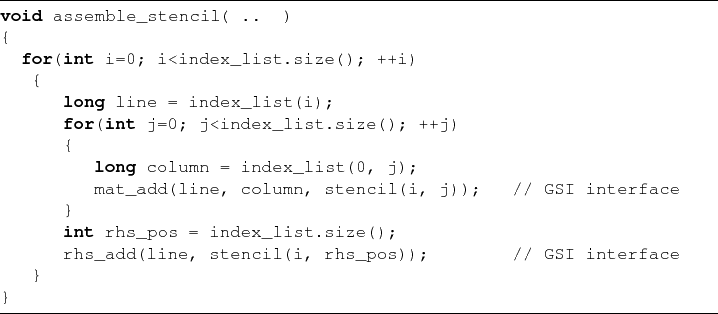Next: 7.4 Performance Analysis Up: 7. A Generic Scientific Previous: 7.2 The Generic Functor

R. Heinzl: Concepts for Scientific Computing